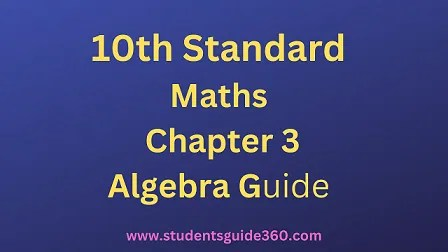# 10th Maths Chapter 3 Algebra Exercise 3.13

10th Standard Maths Chapter 3 Algebra Samacheer Kalvi Guide Exercise 3.13 Book Back Answers Solutions. TN 10th SSLC Samacheer Kalvi Guide. 10th All Subject Guide – Click Here. Class 1 to 12 All Subject Book Back Answers – Click Here## 10th Maths Chapter 3 Algebra Exercise 3.13

### 1. Determine the nature of the roots for the following quadratic equations

• (i) 15.x2 + 11.x + 2 = 0
• (ii) x2 – x – 1 = 0
• (iii) √2t2 – 3t + 3√2= 0
• (iv) 9y2 – 6√2y + 2 = 0
• (v) 9a2b2x2 – 24abcdx + 16c2d2 = 0 a ≠ 0, b ≠ 0

Solution:
(i) 15×2 + 11x + 2 = 0 comparing with ax2 + bx + c = 0.
Here a = 15, 6 = 11, c = 2.
Δ = b2 – 4ac
= 112 -4 × 15 × 2
= 121 – 120
= 1 > 1.
∴ The roots are real and unequal.

(ii) x2 – x – 1 = 0,
Here a = 1, b = -1, c = -1 .
Δ = b2 – 4ac
= (-1)2 – 4 × 1 × -1
= 1 + 4 = 5 > 0.
∴ The roots are real and unequal.

(iii) 2t2 – 3t + 32 = 0

Here a = 2–√, b = -3, c = 32–√
Δ = b2 – 4ac
= (-3)2 – 4 × 2–√ × 32–√
= 9 – 24 = -15 < 0.
∴ The roots are not real.

(iv) 9y2 – 62y + 2 = 0

a = 9, b = 62–√ , c = 2
Δ = b2 – 4ac
= (62–√)2 – 4 × 9 × 2
= 36 × 2 – 72
= 72 – 72 = 0
∴ The roots are real and equal.

(v) 9a2b2x2 – 24abcdx + 16c2d2 = 0
Δ = b2 – 4ac
= (-24abcd)2 – 4 × 9a2b2 × 16c2d2
= 576a2b2c2d2 – 576a2b2c2d2
= 0
∴ The roots are real and equal.

### 2. Find the value(s) of ‘A’ for which the roots of the following equations are real and equal.

(i) (5k – 6)x2 + 2kx + 1 = 0
Here a = 5k – 6 ; b = 2k and c = 1
Since the equation has real and equal roots ∆ = 0.∴ b2 – 4ac = 0
(2k)2 – 4(5k – 6) (1) = 0
4k2 – 20k + 24 = 0
(÷ 4) ⇒ k2 – 5k + 6 = 0
(k – 3) (k – 2) = 0
k -3 = 0 or k – 2 = 0
k = 3 or k = 2
The value of k = 3 or 2

(ii) kx2 + (6k + 2)x + 16 = 0
Here a = k, b = 6k + 2; c = 16
Since the equation has real and equal roots∆ = 0
b2 – 4ac = 0
(6k + 2)2 – 4(k) (16) = 0
36k2 + 4 + 24k – 4(k) (16) = 0
36k2 – 40k + 4 = 0
(÷ by 4) ⇒ 9k2 – 10k + 1 = 0
9k2 – 9k – k + 1 = 0
9k(k – 1) – 1(k – 1) = 0
9k (k – 1) -1 (k – 1) = 0
(k – 1) (9k – 1) = 0
k – 1 or 9k – 1 = 0
k = 1 or k = 19
The value of k = 1 or 19

### 3. If the roots of (a – b)x2 + (b – c)x + (c – a) = 0 are real and equal, then prove that b, a, c are in arithmetic progression.

Solution:
(a – b)x2 + (b – c)x + (c – a) = 0
A = (a – b), B = (b – c), C = (c – a)
Δ = b2 – 4ac = 0
⇒ (b – c)2 – 4(a – b)(c – a)
⇒ b2 – 2bc + c2 -4 (ac – bc – a2 + ab)
⇒ b2 – 2bc + c2 – 4ac + 4bc + 4a2 – 4ab = 0
⇒ 4a2 + b2 + c2 + 2bc – 4ac – 4ab = 0
⇒- (-2a + b + c)2 = 0 [∵ (a + b + c) = a2 + b2 + c2 + 2ab + 2bc + 2ca)]
⇒ 2a + b + c = 0
⇒ 2 a = b + c
∴ a, b, c are in A.P.

### 4. If a, b are real then show that the roots of the equation (a – b)x2 – 6(a + b)x – 9(a – b) = 0 are real and unequal.

(a – b)x2 – 6(a + b)x – 9(a – b) = 0
Here a = a – b ; b = – 6 (a + b); c = – 9 (a – b)
∆ = b2 – 4ac
= [- 6(a + b)]2 – 4(a – b)[-9(a – b)]
= 36(a + b)2 + 36(a – b)(a – b)
= 36 (a + b)2 + 36 (a – b)2
= 36 [(a + b)2 + (a – b)2]
The value is always greater than 0
∆ = 36 [(a + b)2 + (a – b)2] > 0
∴ The roots are real and unequal.

### 5.If the roots of the equation (c2 – ab)x2 – 2(a2 – bc)x + b2 – ac = 0 are real and equal prove that either a = 0 (or) a3 + b3 + c3 = 3abc.

Solution:
(c2 – ab)x2 – 2(a2 – bc)x + (b2 – ac) – 0
Δ = B2 – 4AC = 0 (since the roots are real and equal)
⇒ 4(a2′ – bc)2 – 4 (c2 – ab)(b2 – ac) = 0
⇒ 4(a4 – 2a2bc + b2c2) – 4(c2b2 – ab3 – ac3 + a2bc) = 0
⇒ 4a4 + 4b2c2 – 8a2bc – 4c2b2 + 4ab3 + 4ac3 – 4a2bc = 0
⇒ 4a4+ 4ab3 + 4ac3 – 4a2bc – 8a2bc = 0
⇒ 4a [a3 + b3 + c3] = 0 or a = 0
⇒ a = 0 or [a3 + b3 + c3 – 3abc] = 0
⇒ a3 + b3 + c3 – 3abc = 0
⇒ a3 + b3 + c3 = 3abc or a = 0
Hence proved.